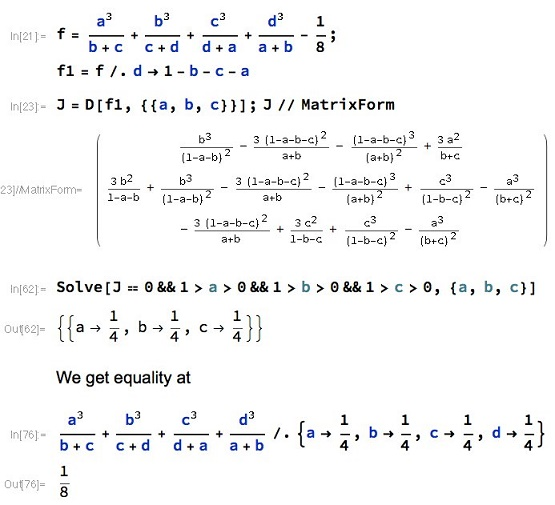# An Inequality with Constraint in Four Variables

### Problem### Solution 1

With Hölder's inequality,

$\displaystyle \left(\sum_{cycl}a\right)^3\le\left(\sum_{cycl}\frac{a^3}{b+c}\right)\left(\sum_{cycl}(b+c)\right)\sum_{cycl}1.$

It follows that

$\displaystyle 1\le\left(\sum_{cycl}\frac{a^3}{b+c}\right)\cdot 2\cdot 4,$

implying the required inequality.

### Solution 2

$\displaystyle\frac{a^3}{b+c}+\frac{b^3}{c+d}+\frac{c^3}{d+a}+\frac{d^3}{a+b}\ge\frac{(a+b+c+d)^3}{\displaystyle\left(\sum_{cycl}\sqrt{b+c}\right)^2}.$

Hence, suffice it to prove that

$\displaystyle\frac{(a+b+c+d)^3}{\displaystyle\left(\sum_{cycl}\sqrt{b+c}\right)^2}\ge\frac{1}{8}.$

Given the constraint, this is equivalent to

(1)

$\displaystyle\left(\sum_{cycl}\sqrt{b+c}\right)^2\le 8.$

Squaring on the left gives

(2)

$\displaystyle\left(\sum_{cycl}\sqrt{b+c}\right)^2 = 2\sum_{cycl}a+2\Pi,$

where $\Pi\,$ is the sum of six pairwise products of the terms $\sqrt{b+c},\,$ $\sqrt{c+d},\,$ $\sqrt{d+a},\,$ $\sqrt{a+b}.\,$ We replace each using the AM-GM inequality, e.g.,

$\displaystyle\sqrt{(b+c)(c+d)}\le\frac{(b+c)+(c+d)}{2},$

each sum on the right containing in the numerator two pairs of the terms $b+c,\,$ $c+d,\,$ $d+a,\,$ $a+b.\,$ Thus there are twelve such pairs in the total that, by symmetry includes equal amounts of each variable. There being four of them, each appears six times in the total. We deduce that $\Pi\le\displaystyle\frac{6(a+b+c+d)}{2}=3(a+b+c+d).$

Returning to (2), we see that (1) will be proved, provided

$\displaystyle 2\sum_{cycl}a+2\Pi \le 2\sum_{cycl}a+6\sum_{cycl}a\le 8.$

But this is true, due to the given constraint.

### Illustration### Acknowledgment

Dan Sitaru has kindly posted the above problem, with a solution, at the CutTheKnotMath facebook page. The problem is by George Apostolopoulos. Solution 1 is by Kevin Soto Palacios. The illustration is by Nassim Nicolas Taleb.

### Inequalities with the Sum of Variables as a Constraint